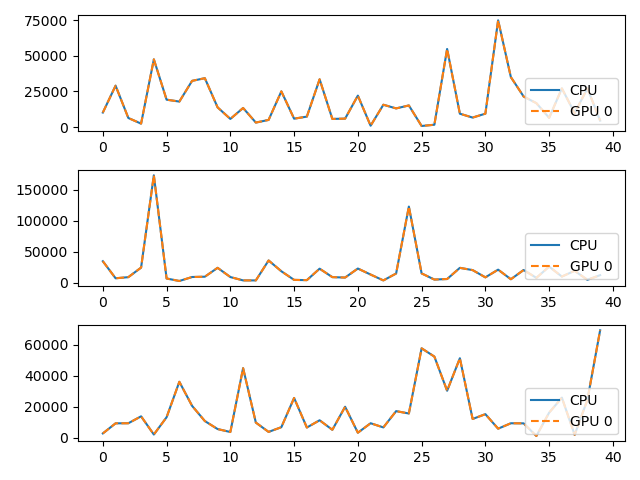# Multi GPU¶

On multi-device clusters, let’s see how to select the card on which a KeOps operation will be performed.

## Setup¶

Standard imports:

import numpy as np
import torch
from matplotlib import pyplot as plt

from pykeops.numpy import Genred


Define the list of gpu ids to be tested:

# By default we assume that there are two GPUs available with 0 and 1 labels:

gpuids = [0,1] if torch.cuda.device_count() > 1 else 


## KeOps Kernel¶

Define some arbitrary KeOps routine:

formula   =  'Square(p-a) * Exp(x+y)'
variables = ['x = Vi(3)','y = Vj(3)','a = Vj(1)','p = Pm(1)']

dtype = 'float32'  # May be 'float32' or 'float64'


## Tests with the NumPy API¶

my_routine = Genred(formula, variables, reduction_op='Sum', axis=1, dtype=dtype)


Generate some data, stored on the CPU (host) memory:

M = 3000
N = 5000
x = np.random.randn(M,3).astype(dtype)
y = np.random.randn(N,3).astype(dtype)
a = np.random.randn(N,1).astype(dtype)
p = np.random.randn(1).astype(dtype)


Launch our routine on the CPU, for reference:

c = my_routine(x, y, a, p, backend='CPU')


And on our GPUs, with copies between the Host and Device memories:

for gpuid in gpuids:
d = my_routine(x, y, a, p, backend='GPU', device_id=gpuid)
print('Relative error on gpu {}: {:1.3e}'.format(gpuid,
float(np.mean( np.abs((c - d) / c)))) )

# Plot the results next to each other:
for i in range(3):
plt.subplot(3, 1, i+1)
plt.plot(c[:40,i],  '-', label='CPU')
plt.plot(d[:40,i], '--', label='GPU {}'.format(gpuid))
plt.legend(loc='lower right')

plt.tight_layout() ; plt.show()Out:

Relative error on gpu 0: 8.562e-07
Relative error on gpu 1: 8.562e-07
/home/bcharlier/keops/pykeops/examples/pytorch/plot_gpu_select_example.py:76: MatplotlibDeprecationWarning:

Adding an axes using the same arguments as a previous axes currently reuses the earlier instance.  In a future version, a new instance will always be created and returned.  Meanwhile, this warning can be suppressed, and the future behavior ensured, by passing a unique label to each axes instance.

/home/bcharlier/keops/pykeops/examples/pytorch/plot_gpu_select_example.py:76: MatplotlibDeprecationWarning:

Adding an axes using the same arguments as a previous axes currently reuses the earlier instance.  In a future version, a new instance will always be created and returned.  Meanwhile, this warning can be suppressed, and the future behavior ensured, by passing a unique label to each axes instance.

/home/bcharlier/keops/pykeops/examples/pytorch/plot_gpu_select_example.py:76: MatplotlibDeprecationWarning:

Adding an axes using the same arguments as a previous axes currently reuses the earlier instance.  In a future version, a new instance will always be created and returned.  Meanwhile, this warning can be suppressed, and the future behavior ensured, by passing a unique label to each axes instance.


## Tests with the PyTorch API¶

import torch
from pykeops.torch import Genred
my_routine = Genred(formula, variables, reduction_op='Sum', axis=1, dtype=dtype)


First, we keep the data on the CPU (host) memory:

x = torch.from_numpy(x)
y = torch.from_numpy(y)
a = torch.from_numpy(a)
p = torch.from_numpy(p)
c = torch.from_numpy(c)

for gpuid in gpuids:
d = my_routine(x, y, a, p, backend='GPU', device_id=gpuid)
print('Relative error on gpu {}: {:1.3e}'.format(gpuid,
float(torch.abs((c - d.cpu()) / c).mean())) )

# Plot the results next to each other:
for i in range(3):
plt.subplot(3, 1, i+1)
plt.plot(c.cpu().numpy()[:40,i],  '-', label='CPU')
plt.plot(d.cpu().numpy()[:40,i], '--', label='GPU {}'.format(gpuid))
plt.legend(loc='lower right')

plt.tight_layout() ; plt.show()Out:

Relative error on gpu 0: 8.562e-07
Relative error on gpu 1: 8.562e-07
/home/bcharlier/keops/pykeops/examples/pytorch/plot_gpu_select_example.py:109: MatplotlibDeprecationWarning:

Adding an axes using the same arguments as a previous axes currently reuses the earlier instance.  In a future version, a new instance will always be created and returned.  Meanwhile, this warning can be suppressed, and the future behavior ensured, by passing a unique label to each axes instance.

/home/bcharlier/keops/pykeops/examples/pytorch/plot_gpu_select_example.py:109: MatplotlibDeprecationWarning:

Adding an axes using the same arguments as a previous axes currently reuses the earlier instance.  In a future version, a new instance will always be created and returned.  Meanwhile, this warning can be suppressed, and the future behavior ensured, by passing a unique label to each axes instance.

/home/bcharlier/keops/pykeops/examples/pytorch/plot_gpu_select_example.py:109: MatplotlibDeprecationWarning:

Adding an axes using the same arguments as a previous axes currently reuses the earlier instance.  In a future version, a new instance will always be created and returned.  Meanwhile, this warning can be suppressed, and the future behavior ensured, by passing a unique label to each axes instance.


Second, we load the data on the GPU (device) of our choice and let KeOps infer the device_id automatically:

for gpuid in gpuids:
with torch.cuda.device(gpuid):
# Transfer the data from Host to Device memory.
# N.B.: The first call to ".cuda()" may take several seconds for each device.
#       This is a known PyTorch issue.
p, a, x, y = p.cuda(), a.cuda(), x.cuda(), y.cuda()

# Call our KeOps routine:
d = my_routine(x, y, a, p, backend='GPU')
print('Relative error on gpu {}: {:1.3e}'.format(gpuid,
float(torch.abs((c - d.cpu()) / c).mean())) )

# Plot the results next to each other:
for i in range(3):
plt.subplot(3, 1, i+1)
plt.plot(c.cpu().numpy()[:40,i],  '-', label='CPU')
plt.plot(d.cpu().numpy()[:40,i], '--', label='GPU {}'.format(gpuid))
plt.legend(loc='lower right')

plt.tight_layout() ; plt.show()Out:

Relative error on gpu 0: 8.562e-07
Relative error on gpu 1: 8.562e-07
/home/bcharlier/keops/pykeops/examples/pytorch/plot_gpu_select_example.py:135: MatplotlibDeprecationWarning:

Adding an axes using the same arguments as a previous axes currently reuses the earlier instance.  In a future version, a new instance will always be created and returned.  Meanwhile, this warning can be suppressed, and the future behavior ensured, by passing a unique label to each axes instance.

/home/bcharlier/keops/pykeops/examples/pytorch/plot_gpu_select_example.py:135: MatplotlibDeprecationWarning:

Adding an axes using the same arguments as a previous axes currently reuses the earlier instance.  In a future version, a new instance will always be created and returned.  Meanwhile, this warning can be suppressed, and the future behavior ensured, by passing a unique label to each axes instance.

/home/bcharlier/keops/pykeops/examples/pytorch/plot_gpu_select_example.py:135: MatplotlibDeprecationWarning:

Adding an axes using the same arguments as a previous axes currently reuses the earlier instance.  In a future version, a new instance will always be created and returned.  Meanwhile, this warning can be suppressed, and the future behavior ensured, by passing a unique label to each axes instance.


Total running time of the script: ( 0 minutes 0.687 seconds)

Gallery generated by Sphinx-Gallery